Search
Document Center

Graph analysis

Last Updated: Aug 17, 2018

Contents

The network analysis column provides analytic algorithms which are based on the Graph data structure. The following figure shows an example of the analysis process developed with the network analysis component of the platform.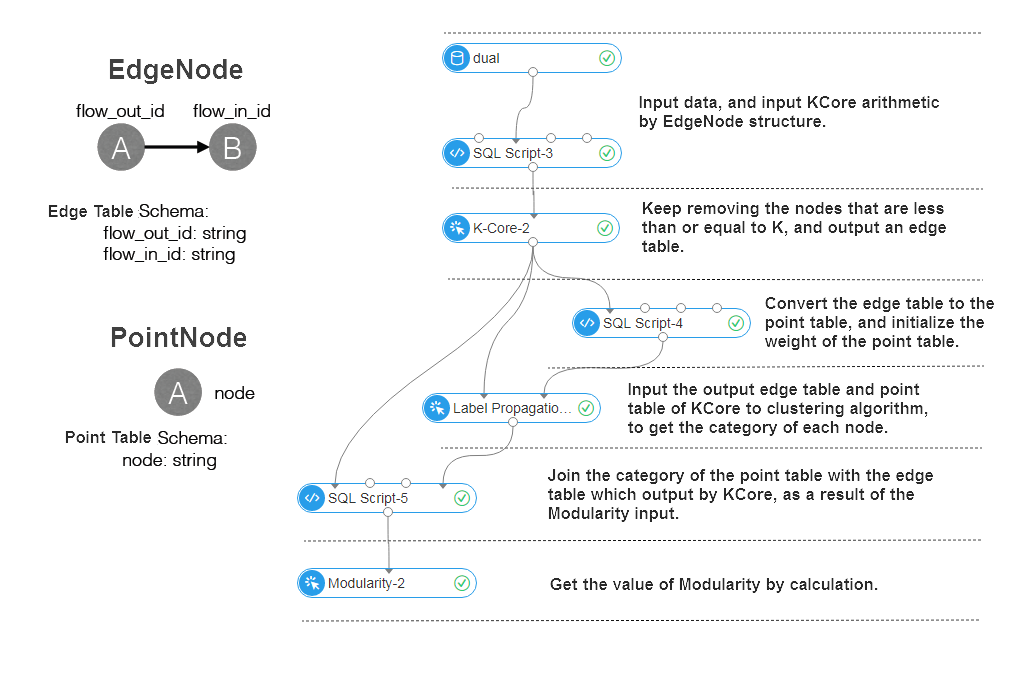The running parameters need to be set for the algorithm components in the network analysis column.
The parameters are described as follows:

• Process count: The workerNum parameter specifies the number of nodes for concurrent job execution. The concurrency level and framework communication costs increase with the value of this parameter.
• Work memory: The workerMem parameter specifies the maximum memory size that a single worker can use. The default value is 4096 MB. The OutOfMemory exception is thrown if memory usage of a single process exceeds the maximum.

k-Core

Function overview

The KCore of a graph is the subgraph that is left after all nodes whose degrees are less than or equal to K are removed. If a node is included in the KCore but is removed from the (K+1)Core, the coreness of this node is K. Therefore, the coreness of a node whose degree is 1 must be 0. The maximum node coreness is the graph coreness.

Parameter settings

K: Coreness value, required, default value is 3.

PAI command

``PAI -name KCore``    -project algo_public``    -DinputEdgeTableName=KCore_func_test_edge``    -DfromVertexCol=flow_out_id``    -DtoVertexCol=flow_in_id``    -DoutputTableName=KCore_func_test_result``    -Dk=2;``

Algorithm parameters

Parameter key Description Required/Optional Default value
inputEdgeTableName Name of the input edge table Required NA
inputEdgeTablePartitions Partitions in the input edge table Optional Entire table
fromVertexCol Start column in the edge table Required NA
toVertexCol End column in the edge table Required NA
outputTableName Name of the output table Required NA
outputTablePartitions Partitions in the output table Optional NA
lifecycle Life cycle of the output table Optional NA
workerNum Worker count Optional Not set
workerMem Worker memory size Optional 4096
splitSize Data split size Optional 64
k Number of cores Required 3

Example

Test data

SQL statement for data generation:

``drop table if exists KCore_func_test_edge;``create table KCore_func_test_edge as``select * from``(``  select '1' as flow_out_id,'2' as flow_in_id from dual``  union all``  select '1' as flow_out_id,'3' as flow_in_id from dual``  union all``  select '1' as flow_out_id,'4' as flow_in_id from dual``  union all``  select '2' as flow_out_id,'3' as flow_in_id from dual``  union all``  select '2' as flow_out_id,'4' as flow_in_id from dual``  union all``  select '3' as flow_out_id,'4' as flow_in_id from dual``  union all``  select '3' as flow_out_id,'5' as flow_in_id from dual``  union all``  select '3' as flow_out_id,'6' as flow_in_id from dual``  union all``  select '5' as flow_out_id,'6' as flow_in_id from dual``)tmp;``

Structure of the graph corresponding to the data:Running result

Set K to 2.
The result is as follows:

``+-------+-------+``| node1 | node2 |``+-------+-------+``| 1     | 2     |``| 1     | 3     |``| 1     | 4     |``| 2     | 1     |``| 2     | 3     |``| 2     | 4     |``| 3     | 1     |``| 3     | 2     |``| 3     | 4     |``| 4     | 1     |``| 4     | 2     |``| 4     | 3     |``+-------+-------+``

Single-source shortest path

Function overview

The single-source shortest path (SSSP) refers to the Dijkstra algorithm. In this algorithm, if the start node is specified, the shortest paths between this node and all other nodes are output.

Parameter settings

Start node ID: ID of the start node used to calculate the shortest paths, which is required.

PAI command

``PAI -name SSSP``    -project algo_public``    -DinputEdgeTableName=SSSP_func_test_edge``    -DfromVertexCol=flow_out_id``    -DtoVertexCol=flow_in_id``    -DoutputTableName=SSSP_func_test_result``    -DhasEdgeWeight=true``    -DedgeWeightCol=edge_weight``    -DstartVertex=a;``

Algorithm parameters

Parameter key Description Required/Optional Default value
inputEdgeTableName Name of the input edge table Required NA
inputEdgeTablePartitions Partitions in the input edge table Optional Entire table
fromVertexCol Start column in the input edge table Required NA
toVertexCol End column in the input edge table Required NA
outputTableName Name of the output table Required NA
outputTablePartitions Partitions in the output table Optional NA
lifecycle Life cycle of the output table Optional NA
workerNum Worker count Optional Not set
workerMem Worker memory size Optional 4096
splitSize Data split size Optional 64
startVertex Start node ID Required NA
hasEdgeWeight Indicates whether the edge of the input edge table has weight Optional false
edgeWeightCol Edge weight column in the input edge table Optional NA

Example

Test data

SQL statement for data generation:

``drop table if exists SSSP_func_test_edge;``create table SSSP_func_test_edge as``select``    flow_out_id,flow_in_id,edge_weight``from``(``    select "a" as flow_out_id,"b" as flow_in_id,1.0 as edge_weight from dual``    union all``    select "b" as flow_out_id,"c" as flow_in_id,2.0 as edge_weight from dual``    union all``    select "c" as flow_out_id,"d" as flow_in_id,1.0 as edge_weight from dual``    union all``    select "b" as flow_out_id,"e" as flow_in_id,2.0 as edge_weight from dual``    union all``    select "e" as flow_out_id,"d" as flow_in_id,1.0 as edge_weight from dual``    union all``    select "c" as flow_out_id,"e" as flow_in_id,1.0 as edge_weight from dual``    union all``    select "f" as flow_out_id,"g" as flow_in_id,3.0 as edge_weight from dual``    union all``    select "a" as flow_out_id,"d" as flow_in_id,4.0 as edge_weight from dual``) tmp;``

Structure of the graph corresponding to the data: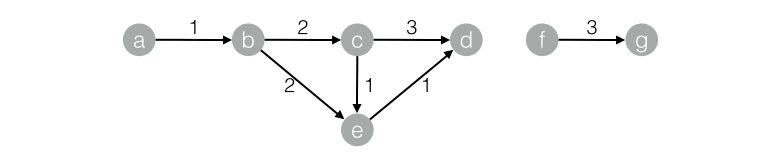Running result

``+------------+------------+------------+--------------+``| start_node | dest_node  | distance   | distance_cnt | ``+------------+------------+------------+--------------+``| a          | b          | 1.0        | 1            |``| a          | c          | 3.0        | 1            |``| a          | d          | 4.0        | 3            |``| a          | a          | 0.0        | 0            |``| a          | e          | 3.0        | 1            |``+------------+------------+------------+--------------+``

PageRank

Function overview

PageRank is an algorithm used by Google Search to rank websites based on the structure of links to a page. The underlying assumption is as follows:

• More important or quality websites are likely to receive more links from other websites.

• Besides the quantity of links to a web page, the weight of the web page and the number of outgoing links also count in the calculation.

• For the social networks of a user, the edge weight is an important factor in addition to the influence of the user.

For example, a Sina Weibo user is more likely to have influence on the family, friends, classmates, and colleagues among the user’s followers, while less on the followers of weak relationship such as strangers. In social networks, the edge weight is equivalent to the user-user relationship strength index.

The PageRank formula with the link weight is as follows: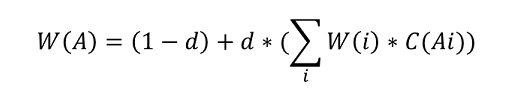• W(i): The weight of node i.
• d: The damping factor.
• W(A): The node weight after the algorithm iteration is stable, which is the influence index of each user.

Parameter settings

Maximum number of iterations: (Optional) The number of iterations before the algorithm automatically converges. The default value is 30.

PAI command

``PAI -name PageRankWithWeight``    -project algo_public``    -DinputEdgeTableName=PageRankWithWeight_func_test_edge``    -DfromVertexCol=flow_out_id``    -DtoVertexCol=flow_in_id``    -DoutputTableName=PageRankWithWeight_func_test_result``    -DhasEdgeWeight=true``    -DedgeWeightCol=weight``    -DmaxIter 100;``

Algorithm parameters

Parameter key Description Required/Optional Default value
inputEdgeTableName Name of the input edge table Required NA
inputEdgeTablePartitions Partitions in the input edge table Optional Entire table
fromVertexCol Start column in the input edge table Required NA
toVertexCol End column in the input edge table Required NA
outputTableName Name of the output table Required NA
outputTablePartitions Partitions in the output table Optional NA
lifecycle Life cycle of the output table Optional NA
workerNum Worker count Optional Not set
workerMem Worker memory size Optional 4096
splitSize Data split size Optional 64
hasEdgeWeight Indicates whether the edge of the input edge table has weight Optional false
edgeWeightCol Edge weight column in the input edge table Optional NA
maxIter Maximum number of iterations Optional 30

Example

Test data

SQL statement for data generation:

``drop table if exists PageRankWithWeight_func_test_edge;``create table PageRankWithWeight_func_test_edge as``select * from``(``    select 'a' as flow_out_id,'b' as flow_in_id,1.0 as weight from dual``    union all``    select 'a' as flow_out_id,'c' as flow_in_id,1.0 as weight from dual``    union all``    select 'b' as flow_out_id,'c' as flow_in_id,1.0 as weight from dual``    union all``    select 'b' as flow_out_id,'d' as flow_in_id,1.0 as weight from dual``    union all``    select 'c' as flow_out_id,'d' as flow_in_id,1.0 as weight from dual``)tmp;``

Structure of the graph corresponding to the data:Running result

``+------+------------+``| node | weight     |``+------+------------+``| a    | 0.0375     |``| b    | 0.06938    |``| c    | 0.12834    |``| d    | 0.20556    |``+------+------------+``

Label propagation clustering

Function overview

Graph clustering is used to divide subgraphs based on the graph topology such that the links between the nodes in the subgraphs are more than those between subgraphs. The label propagation algorithm (LPA) is a graph-based semi-supervised machine learning algorithm. The labels of a node (community) depend on those of the neighboring nodes. The dependence degree is determined by the similarity between nodes, and data becomes stable by iterative propagation update.

Parameter settings

Maximum number of iterations: (Optional) The default value is 30.

PAI command

``PAI -name LabelPropagationClustering``    -project algo_public``    -DinputEdgeTableName=LabelPropagationClustering_func_test_edge``    -DfromVertexCol=flow_out_id``    -DtoVertexCol=flow_in_id``    -DinputVertexTableName=LabelPropagationClustering_func_test_node``    -DvertexCol=node``    -DoutputTableName=LabelPropagationClustering_func_test_result``    -DhasEdgeWeight=true``    -DedgeWeightCol=edge_weight``    -DhasVertexWeight=true``    -DvertexWeightCol=node_weight``    -DrandSelect=true``    -DmaxIter=100;``

Algorithm parameters

Parameter key Description Required/Optional Default value
inputEdgeTableName Name of the input edge table Required NA
inputEdgeTablePartitions Partitions in the input edge table Optional Entire table
fromVertexCol Start column in the input edge table Required NA
toVertexCol End column in the input edge table Required NA
inputVertexTableName Name of the input vertex table Required NA
inputVertexTablePartitions Partitions in the input vertex table Optional Entire table
vertexCol Vertex column in the input vertex table Required NA
outputTableName Name of the output table Required NA
outputTablePartitions Partitions in the output table Optional NA
lifecycle Life cycle of the output table Optional NA
workerNum Worker count Optional Not set
workerMem Worker memory size Optional 4096
splitSize Data split size Optional 64
hasEdgeWeight Indicates whether the edge of the input edge table has weight Optional false
edgeWeightCol Edge weight column in the input edge table Optional NA
hasVertexWeight Indicates whether the vertexes of the input vertex table have weights Optional false
vertexWeightCol Vertex weight column in the input vertex table Optional NA
randSelect Indicates whether the maximum label is randomly selected Optional false
maxIter Maximum number of iterations Optional 30

Example

Test data

SQL statement for data generation:

``drop table if exists LabelPropagationClustering_func_test_edge;``create table LabelPropagationClustering_func_test_edge as``select * from``(``    select '1' as flow_out_id,'2' as flow_in_id,0.7 as edge_weight from dual``    union all``    select '1' as flow_out_id,'3' as flow_in_id,0.7 as edge_weight from dual``    union all``    select '1' as flow_out_id,'4' as flow_in_id,0.6 as edge_weight from dual``    union all``    select '2' as flow_out_id,'3' as flow_in_id,0.7 as edge_weight from dual``    union all``    select '2' as flow_out_id,'4' as flow_in_id,0.6 as edge_weight from dual``    union all``    select '3' as flow_out_id,'4' as flow_in_id,0.6 as edge_weight from dual``    union all``    select '4' as flow_out_id,'6' as flow_in_id,0.3 as edge_weight from dual``    union all``    select '5' as flow_out_id,'6' as flow_in_id,0.6 as edge_weight from dual``    union all``    select '5' as flow_out_id,'7' as flow_in_id,0.7 as edge_weight from dual``    union all``    select '5' as flow_out_id,'8' as flow_in_id,0.7 as edge_weight from dual``    union all``    select '6' as flow_out_id,'7' as flow_in_id,0.6 as edge_weight from dual``    union all``    select '6' as flow_out_id,'8' as flow_in_id,0.6 as edge_weight from dual``    union all``    select '7' as flow_out_id,'8' as flow_in_id,0.7 as edge_weight from dual``)tmp;``drop table if exists LabelPropagationClustering_func_test_node;``create table LabelPropagationClustering_func_test_node as``select * from``(``    select '1' as node,0.7 as node_weight from dual``    union all``    select '2' as node,0.7 as node_weight from dual``    union all``    select '3' as node,0.7 as node_weight from dual``    union all``    select '4' as node,0.5 as node_weight from dual``    union all``    select '5' as node,0.7 as node_weight from dual``    union all``    select '6' as node,0.5 as node_weight from dual``    union all``    select '7' as node,0.7 as node_weight from dual``    union all``    select '8' as node,0.7 as node_weight from dual``)tmp;``

Structure of the group corresponding to the data: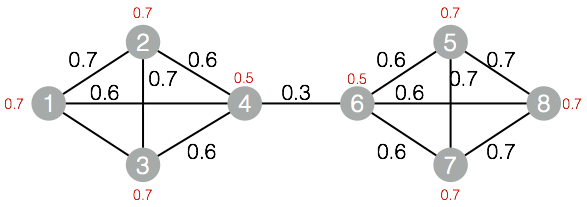Running result

``+------+------------+``| node | group_id   |``+------+------------+``| 1    | 1          |``| 2    | 1          |``| 3    | 1          |``| 4    | 1          |``| 5    | 5          |``| 6    | 5          |``| 7    | 5          |``| 8    | 5          |``+------+------------+``

Label propagation classification

Function overview

Label propagation classification is a semi-supervised classification algorithm that uses the label information of labeled nodes to predict that of unlabeled nodes.

During algorithm execution, the labels of each node are propagated to the neighboring nodes based on the similarity between the nodes. In each step of propagation, a node updates its label based on the labels of the neighboring nodes such that the node is more similar with the neighboring nodes. The higher the similarity, the greater weight the neighboring nodes have on that node, and the easier for labels to be propagated. During label propagation, the labels of the labeled data remain unchanged, which serve as sources for propagation to the unlabeled data.

After the iterations end, the probability distributions of similar nodes are also similar such that the nodes can be divided into the same category to complete label propagation.

Parameter settings

• Damping factor: The default value is 0.8.
• Convergence factor: The default value is 0.000001.

PAI command

``PAI -name LabelPropagationClassification``    -project algo_public``    -DinputEdgeTableName=LabelPropagationClassification_func_test_edge``    -DfromVertexCol=flow_out_id``    -DtoVertexCol=flow_in_id``    -DinputVertexTableName=LabelPropagationClassification_func_test_node``    -DvertexCol=node``    -DvertexLabelCol=label``    -DoutputTableName=LabelPropagationClassification_func_test_result``    -DhasEdgeWeight=true``    -DedgeWeightCol=edge_weight``    -DhasVertexWeight=true``    -DvertexWeightCol=label_weight``    -Dalpha=0.8``    -Depsilon=0.000001;``

Algorithm parameters

Parameter key Description Required/Optional Default value
inputEdgeTableName Name of the input edge table Required NA
inputEdgeTablePartitions Partitions in the input edge table Optional Entire table
fromVertexCol Start column in the input edge table Required NA
toVertexCol End column in the input edge table Required NA
inputVertexTableName Name of the input vertex table Required NA
inputVertexTablePartitions Partitions in the input vertex table Optional Entire table
vertexCol Vertex column in the input vertex table Required NA
vertexLabelCol Vertex label column in the input vertex table Required NA
outputTableName Name of the output table Required NA
outputTablePartitions Partitions in the output table Optional NA
lifecycle Life cycle of the output table Optional NA
workerNum Worker count Optional Not set
workerMem Worker memory size Optional 4096
splitSize Data split size Optional 64
hasEdgeWeight Indicates whether the edge of the input edge table has weight Optional false
edgeWeightCol Edge weight column in the input edge table Optional NA
hasVertexWeight Indicates whether the vertexes of the input vertex table have weights Optional false
vertexWeightCol Vertex weight column in the input vertex table Optional NA
alpha Damping factor Optional 0.8
epsilon Convergence factor Optional 0.000001
maxIter Maximum number of iterations Optional 30

Example

Test data

SQL statement for data generation:

``drop table if exists LabelPropagationClassification_func_test_edge;``create table LabelPropagationClassification_func_test_edge as``select * from``(``    select 'a' as flow_out_id, 'b' as flow_in_id, 0.2 as edge_weight from dual``    union all``    select 'a' as flow_out_id, 'c' as flow_in_id, 0.8 as edge_weight from dual``    union all``    select 'b' as flow_out_id, 'c' as flow_in_id, 1.0 as edge_weight from dual``    union all``    select 'd' as flow_out_id, 'b' as flow_in_id, 1.0 as edge_weight from dual``)tmp;``drop table if exists LabelPropagationClassification_func_test_node;``create table LabelPropagationClassification_func_test_node as``select * from``(``    select 'a' as node,'X' as label, 1.0 as label_weight from dual``    union all``    select 'd' as node,'Y' as label, 1.0 as label_weight from dual``)tmp;``

Structure of the graph corresponding to the data: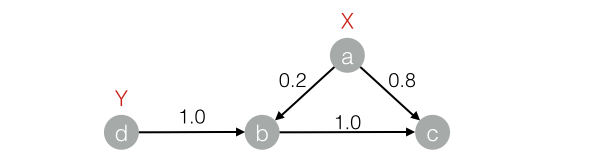Running result

``+------+-----+------------+``| node | tag | weight     |``+------+-----+------------+``| a    | X   | 1.0        |``| b    | X   | 0.16667    |``| b    | Y   | 0.83333    |``| c    | X   | 0.53704    |``| c    | Y   | 0.46296    |``| d    | Y   | 1.0        |``+------+-----+------------+``

Modularity

Function overview

Modularity is a measure of the structure of networks. It measures the closeness of communities divided from a network structure. A value larger than 0.3 represents an obvious community structure.

PAI command

``PAI -name Modularity``    -project algo_public``    -DinputEdgeTableName=Modularity_func_test_edge``    -DfromVertexCol=flow_out_id``    -DfromGroupCol=group_out_id``    -DtoVertexCol=flow_in_id``    -DtoGroupCol=group_in_id``    -DoutputTableName=Modularity_func_test_result;``

Algorithm parameters

Parameter key Description Required/Optional Default value
inputEdgeTableName Name of the input edge table Required NA
inputEdgeTablePartitions Partitions in the input edge table Optional Entire table
fromVertexCol Start column in the input edge table Required NA
fromGroupCol Group of the start node in the input edge table Required NA
toVertexCol End column in the input edge table Required NA
toGroupCol Group of the end vertex in the input edge table Required NA
outputTableName Name of the output table Required NA
outputTablePartitions Partitions in the output table Optional NA
lifecycle Life cycle of the output table Optional NA
workerNum Worker count Optional Not set
workerMem Worker memory size Optional 4096
splitSize Data split size Optional 64

Example

Test data

Same as the data in Label propagation clustering.

Running result

``+--------------+``| val          |``+--------------+``| 0.4230769    |``+--------------+``

Maximum connected subgraphs

Function overview

In an undirected graph G, vertex A is connected to vertex B if a path exists between them. Graph G contains several subgraphs, where each vertex is connected to every other vertex in the same subgraph but is separated from those in other subgraphs. These subgraphs of graph G are maximum connected subgraphs.

None

PAI command

``PAI -name MaximalConnectedComponent ``    -project algo_public ``    -DinputEdgeTableName=MaximalConnectedComponent_func_test_edge ``    -DfromVertexCol=flow_out_id``    -DtoVertexCol=flow_in_id ``    -DoutputTableName=MaximalConnectedComponent_func_test_result;``

Algorithm parameters

Parameter key Description Required/Optional Default value
inputEdgeTableName Name of the input edge table Required NA
inputEdgeTablePartitions Partitions in the input edge table Optional Entire table
fromVertexCol Start column in the input edge table Required NA
toVertexCol End column in the input edge table Required NA
outputTableName Name of the output table Required NA
outputTablePartitions Partitions in the output table Optional NA
lifecycle Life cycle of the output table Optional NA
workerNum Worker count Optional Not set
workerMem Worker memory size Optional 4096
splitSize Data split size Optional 64

Example

Test data

SQL statement for data generation:

``drop table if exists MaximalConnectedComponent_func_test_edge;``create table MaximalConnectedComponent_func_test_edge as``select * from``(``  select '1' as flow_out_id,'2' as flow_in_id from dual``  union all``  select '2' as flow_out_id,'3' as flow_in_id from dual``  union all``  select '3' as flow_out_id,'4' as flow_in_id from dual``  union all``  select '1' as flow_out_id,'4' as flow_in_id from dual``  union all``  select 'a' as flow_out_id,'b' as flow_in_id from dual``  union all``  select 'b' as flow_out_id,'c' as flow_in_id from dual``)tmp;``drop table if exists MaximalConnectedComponent_func_test_result;``create table MaximalConnectedComponent_func_test_result``(``  node string,``  grp_id string``);``

Structure of the graph corresponding to the data:Running result

``+-------+-------+``| node  | grp_id|``+-------+-------+``| 1     | 4     |``| 2     | 4     |``| 3     | 4     |``| 4     | 4     |``| a     | c     |``| b     | c     |``| c     | c     |``+-------+-------+``

Node clustering coefficient

Function overview

This coefficient is used to calculate the peripheral density of a node in an undirected graph G. The density of a star network is 0, and that of a fully meshed network is 1.

Parameter settings

maxEdgeCnt: If the node degree is greater than the value of this parameter, sampling is performed. This parameter is optional, and the default value is 500.

PAI command

``PAI -name NodeDensity``    -project algo_public``    -DinputEdgeTableName=NodeDensity_func_test_edge``    -DfromVertexCol=flow_out_id``    -DtoVertexCol=flow_in_id``    -DoutputTableName=NodeDensity_func_test_result``    -DmaxEdgeCnt=500;``

Algorithm parameters

Parameter key Description Required/Optional Default value
inputEdgeTableName Name of the input edge table Required NA
inputEdgeTablePartitions Partitions in the input edge table Optional Entire table
fromVertexCol Start column in the input edge table Required NA
toVertexCol End column in the input edge table Required NA
outputTableName Name of the output table Required NA
outputTablePartitions Partitions in the output table Optional NA
lifecycle Life cycle of the output table Optional NA
maxEdgeCnt If the node degree is greater than the value of this parameter, sampling is performed. Optional 500
workerNum Worker count Optional Not set
workerMem Worker memory size Optional 4096
splitSize Data split size Optional 64

Example

Test data

SQL statement for data generation:

``drop table if exists NodeDensity_func_test_edge;``create table NodeDensity_func_test_edge as``select * from``(``  select '1' as flow_out_id, '2' as flow_in_id from dual``  union all``  select '1' as flow_out_id, '3' as flow_in_id from dual``  union all``  select '1' as flow_out_id, '4' as flow_in_id from dual``  union all``  select '1' as flow_out_id, '5' as flow_in_id from dual``  union all``  select '1' as flow_out_id, '6' as flow_in_id from dual``  union all``  select '2' as flow_out_id, '3' as flow_in_id from dual``  union all``  select '3' as flow_out_id, '4' as flow_in_id from dual``  union all``  select '4' as flow_out_id, '5' as flow_in_id from dual``  union all``  select '5' as flow_out_id, '6' as flow_in_id from dual``  union all``  select '5' as flow_out_id, '7' as flow_in_id from dual``  union all``  select '6' as flow_out_id, '7' as flow_in_id from dual``)tmp;``drop table if exists NodeDensity_func_test_result;``create table NodeDensity_func_test_result``(``  node string,``  node_cnt bigint,``  edge_cnt bigint,``  density double,``  log_density double``);``

Structure of the graph corresponding to the data:Running result

``1,5,4,0.4,1.45657``2,2,1,1.0,1.24696``3,3,2,0.66667,1.35204``4,3,2,0.66667,1.35204``5,4,3,0.5,1.41189``6,3,2,0.66667,1.35204``7,2,1,1.0,1.24696``

Edge clustering coefficient

Function overview

This coefficient is used to calculate the peripheral density of each edge in an undirected graph G.

None

PAI command

``PAI -name EdgeDensity``    -project algo_public``    -DinputEdgeTableName=EdgeDensity_func_test_edge``    -DfromVertexCol=flow_out_id``    -DtoVertexCol=flow_in_id``    -DoutputTableName=EdgeDensity_func_test_result;``

Algorithm parameters

Parameter key Description Required/Optional Default value
inputEdgeTableName Name of the input edge table Required NA
inputEdgeTablePartitions Partitions in the input edge table Optional Entire table
fromVertexCol Start column in the input edge table Required NA
toVertexCol End column in the input edge table Required NA
outputTableName Name of the output table Required NA
outputTablePartitions Partitions in the output table Optional NA
lifecycle Life cycle of the output table Optional NA
workerNum Worker count Optional Not set
workerMem Worker memory size Optional 4096
splitSize Data split size Optional 64

Example

Test data

SQL statement for data generation:

``drop table if exists EdgeDensity_func_test_edge;``create table EdgeDensity_func_test_edge as``select * from``(``  select '1' as flow_out_id,'2' as flow_in_id from dual``  union all``  select '1' as flow_out_id,'3' as flow_in_id from dual``  union all``  select '1' as flow_out_id,'5' as flow_in_id from dual``  union all``  select '1' as flow_out_id,'7' as flow_in_id from dual``  union all``  select '2' as flow_out_id,'5' as flow_in_id from dual``  union all``  select '2' as flow_out_id,'4' as flow_in_id from dual``  union all``  select '2' as flow_out_id,'3' as flow_in_id from dual``  union all``  select '3' as flow_out_id,'5' as flow_in_id from dual``  union all``  select '3' as flow_out_id,'4' as flow_in_id from dual``  union all``  select '4' as flow_out_id,'5' as flow_in_id from dual``  union all``  select '4' as flow_out_id,'8' as flow_in_id from dual``  union all``  select '5' as flow_out_id,'6' as flow_in_id from dual``  union all``  select '5' as flow_out_id,'7' as flow_in_id from dual``  union all``  select '5' as flow_out_id,'8' as flow_in_id from dual``  union all``  select '7' as flow_out_id,'6' as flow_in_id from dual``  union all``  select '6' as flow_out_id,'8' as flow_in_id from dual``)tmp;``drop table if exists EdgeDensity_func_test_result;``create table EdgeDensity_func_test_result``(``  node1 string,``  node2 string,``  node1_edge_cnt bigint,``  node2_edge_cnt bigint,``  triangle_cnt bigint,``  density double``);``

Structure of the graph corresponding to the data:Running result

``1,2,4,4,2,0.5``2,3,4,4,3,0.75``2,5,4,7,3,0.75``3,1,4,4,2,0.5``3,4,4,4,2,0.5``4,2,4,4,2,0.5``4,5,4,7,3,0.75``5,1,7,4,3,0.75``5,3,7,4,3,0.75``5,6,7,3,2,0.66667``5,8,7,3,2,0.66667``6,7,3,3,1,0.33333``7,1,3,4,1,0.33333``7,5,3,7,2,0.66667``8,4,3,4,1,0.33333``8,6,3,3,1,0.33333``

Triangle count

Function overview

Output all triangles in an undirected graph G.

Parameter settings

maxEdgeCnt: If the node degree is greater than the value of this parameter, sampling is performed. This parameter is optional, and the default value is 500.

PAI command

``PAI -name TriangleCount``    -project algo_public``    -DinputEdgeTableName=TriangleCount_func_test_edge``    -DfromVertexCol=flow_out_id``    -DtoVertexCol=flow_in_id``    -DoutputTableName=TriangleCount_func_test_result;``

Algorithm parameters

Parameter key Description Required/Optional Default value
inputEdgeTableName Name of the input edge table Required NA
inputEdgeTablePartitions Partitions in the input edge table Optional Entire table
fromVertexCol Start column in the input edge table Required NA
toVertexCol End column in the input edge table Required NA
outputTableName Name of the output table Required NA
outputTablePartitions Partitions in the output table Optional NA
lifecycle Life cycle of the output table Optional NA
maxEdgeCnt If the node degree is greater than the value of this parameter, sampling is performed. Optional 500
workerNum Worker count Optional Not set
workerMem Worker memory size Optional 4096
splitSize Data split size Optional 64

Example

Test data

SQL statement for data generation:

``drop table if exists TriangleCount_func_test_edge;``create table TriangleCount_func_test_edge as``select * from``(``  select '1' as flow_out_id,'2' as flow_in_id from dual``  union all``  select '1' as flow_out_id,'3' as flow_in_id from dual``  union all``  select '1' as flow_out_id,'4' as flow_in_id from dual``  union all``  select '1' as flow_out_id,'5' as flow_in_id from dual``  union all``  select '1' as flow_out_id,'6' as flow_in_id from dual``  union all``  select '2' as flow_out_id,'3' as flow_in_id from dual``  union all``  select '3' as flow_out_id,'4' as flow_in_id from dual``  union all``  select '4' as flow_out_id,'5' as flow_in_id from dual``  union all``  select '5' as flow_out_id,'6' as flow_in_id from dual``  union all``  select '5' as flow_out_id,'7' as flow_in_id from dual``  union all``  select '6' as flow_out_id,'7' as flow_in_id from dual``)tmp;``drop table if exists TriangleCount_func_test_result;``create table TriangleCount_func_test_result``(``  node1 string,``  node2 string,``  node3 string``);``

Structure of the graph corresponding to the data:Running result

``1,2,3``1,3,4``1,4,5``1,5,6``5,6,7``

Decision tree depth

Function overview

Output depth and tree ID of each node in a network composed of many trees.

None

PAI command

``PAI -name TreeDepth``    -project algo_public``    -DinputEdgeTableName=TreeDepth_func_test_edge``    -DfromVertexCol=flow_out_id``    -DtoVertexCol=flow_in_id``    -DoutputTableName=TreeDepth_func_test_result;``

Algorithm parameters

Parameter key Description Required/Optional Default value
inputEdgeTableName Name of the input edge table Required NA
inputEdgeTablePartitions Partitions in the input edge table Optional Entire table
fromVertexCol Start column in the input edge table Required NA
toVertexCol End column in the input edge table Required NA
outputTableName Name of the output table Required NA
outputTablePartitions Partitions in the output table Optional NA
lifecycle Life cycle of the output table Optional NA
workerNum Worker count Optional Not set
workerMem Worker memory size Optional 4096
splitSize Data split size Optional 64

Example

Test data

SQL statement for data generation:

``drop table if exists TreeDepth_func_test_edge;``create table TreeDepth_func_test_edge as``select * from``(``    select '0' as flow_out_id, '1' as flow_in_id from dual``    union all``    select '0' as flow_out_id, '2' as flow_in_id from dual``    union all``    select '1' as flow_out_id, '3' as flow_in_id from dual``    union all``    select '1' as flow_out_id, '4' as flow_in_id from dual``    union all``    select '2' as flow_out_id, '4' as flow_in_id from dual``    union all``    select '2' as flow_out_id, '5' as flow_in_id from dual``    union all``    select '4' as flow_out_id, '6' as flow_in_id from dual``    union all``    select 'a' as flow_out_id, 'b' as flow_in_id from dual``    union all``    select 'a' as flow_out_id, 'c' as flow_in_id from dual``    union all``    select 'c' as flow_out_id, 'd' as flow_in_id from dual``    union all``    select 'c' as flow_out_id, 'e' as flow_in_id from dual``)tmp;``drop table if exists TreeDepth_func_test_result;``create table TreeDepth_func_test_result``(``  node string,``  root string,``  depth bigint``);``

Structure of the graph corresponding to the data:Running result

``0,0,0``1,0,1``2,0,1``3,0,2``4,0,2``5,0,2``6,0,3``a,a,0``b,a,1``c,a,1``d,a,2``e,a,2``